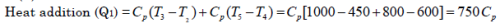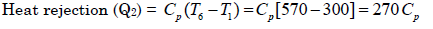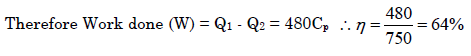Courses

# Gas Turbine - MCQ Test 2

## 30 Questions MCQ Test RRB JE for Mechanical Engineering | Gas Turbine - MCQ Test 2

Description
This mock test of Gas Turbine - MCQ Test 2 for Mechanical Engineering helps you for every Mechanical Engineering entrance exam. This contains 30 Multiple Choice Questions for Mechanical Engineering Gas Turbine - MCQ Test 2 (mcq) to study with solutions a complete question bank. The solved questions answers in this Gas Turbine - MCQ Test 2 quiz give you a good mix of easy questions and tough questions. Mechanical Engineering students definitely take this Gas Turbine - MCQ Test 2 exercise for a better result in the exam. You can find other Gas Turbine - MCQ Test 2 extra questions, long questions & short questions for Mechanical Engineering on EduRev as well by searching above.
QUESTION: 1

### An open cycle constant pressure gas turbine uses a fuel of calorific value 40,000 kJ/kg, with air fuel ratio of 80:1 and develops a net output of 80 kJ/kg of air. What is the thermal efficiency of the cycle?

Solution:

take air = 80 kg so fuel = 1 kg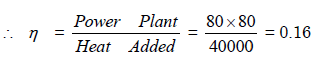QUESTION: 2

### . In a gas turbine cycle, the turbine output is 600kJ/kg, the compressor work is 400kJ/kg and the heat supplied is 1000 kJ/kg. The thermal efficiency of this cycle is:

Solution: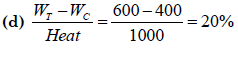QUESTION: 3

### The power required to drive a turbo-compressor for a given pressure ratio decreases when

Solution:
QUESTION: 4

Optimum pressure ratio for maximum specific output for ideal gas turbine plant operating at initial temperature of 300 k and maximum temperature of 100 k is closer to

Solution:

Maximum possible pressure ratio, (Rr)max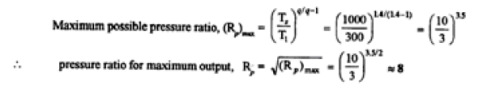QUESTION: 5

In a single-stage open-cycle gas turbine, the mass flow through the turbine is higher than the mass flow through compressor, because

Solution:

Due to combustion of fuel in combustion chamber, mass flow through turbine is higher than compressor

QUESTION: 6

The given figure shows the effect of the substitution of an isothermal compression process for the isentropic compression process onthe gas turbine cycle. The shadedare (1-5-2-1) in the p-v diagramr epresents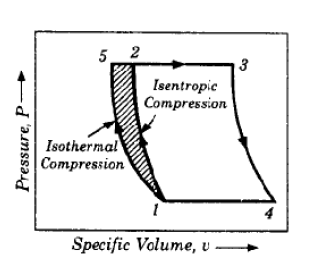Solution:

Shaded area corresponds to increment in the compression work

QUESTION: 7

Which one of the following statements is correct ? Increasing the number of reheating stages a gas turbine to infinity, makesthe expansion tending:

Solution:

• Increasing the number of Reheating stages in a gas turbine to infinity makes the expansion tending to isothermal.
• Increasing the intercooling in compressor to infinity also makes the compression tending to isothermal.
• This is known as Erecessionisation of the Brayton cycle

QUESTION: 8

Which one of the following is correct ? In a gas turbine cycle with regeneration,

Solution:
QUESTION: 9

The efficiency of a simple gas turbine can be improved by using are generator, because the

Solution:
QUESTION: 10

A gas turbine plant working on Joule cycle produces 4000 kW of power. Ifits work ratio is 40%, what is the power consumed by the compressor?

Solution: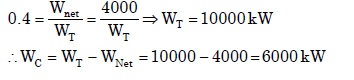QUESTION: 11

A gas turbine develops 120 kJ of work while the compressor absorbed 60kJ of work and the heat supplied is 100 kJ. If a regenerator which wouldrecover 40% of the heat in the exhaust was used, then the increase in theoverall thermal efficiency would be:

Solution: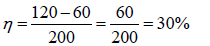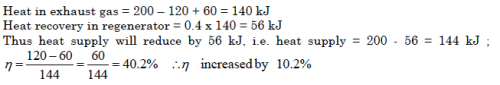QUESTION: 12

The use of regenerator in a gas turbine cycle

Solution:

The addition of regenerator in gas turbine reduces the heat required from
external source but work output remains same and efficiency increases.

QUESTION: 13

Consider the following statements in respect of gas turbines: A gas turbine plant with reheater leads to a1. Considerable improvement in the work output2. Considerable improvement in the thermal efficiency.

Solution:

In gas power plant reheat always decrease thermal efficiency

QUESTION: 14

Assertion (A): In a gas turbine, reheating is preferred over regeneration to yield ahigher thermal efficiency.

Reason (R): The thermal efficiency given by the ratio of the difference of work doneby turbine (Wt) and work required by compressor (Wc) to heat added (QA) isimproved by increasing the Wt keeping Wc and QA constant in reheating, whereas inregeneration QA is reduced keeping Wt and Wc constant.

Solution:

A is false but R is true

QUESTION: 15

Reheating in a gas turbine

Solution:
QUESTION: 16

Inter-cooling in gas turbines

Solution:

Inter-cooling in gas turbine is used to compress air in two stages with intercooling. With inter-cooling the work to be done on compressor decreases and thus net output of turbine increases. However more heat has to be added in combustion chamber which results in decrease in thermal efficiency.

QUESTION: 17

Consider the following statements:

1. Inter-cooling is effective only at lower pressure ratios and high turbineinlet temperatures

2. There is very little gain in thermal efficiency when inter-cooling is usedwithout the benefit of regeneration

3. With high values of 'γ' and cp of the working fluid, the net power outputof Brayton cycle will increase.

Solution:
QUESTION: 18

Consider the following statements: When air is to be compressed to reasonably high pressure, it is usually carried out by a multistage compressor with an intercooler between thestages because

1. Work supplied is saved.

2. Weight of compressor is reduced.

3. More uniform torque is obtained leading to the reduction in the size offlywheel.

4. Volumetric efficiency is increased.Of the four statements listed above correct is/are?

Solution:
QUESTION: 19

Consider the following statements: Which of the following increase the work ratio in a simple gas turbine plant ?

1. Heat exchanger

2. Inter cooling

3. Reheating Select the correct answer using the code given below:

Solution:
QUESTION: 20

Assertion (A): In gas turbines, regenerative heating always improves the efficiency unlike that in the case of reheating.

Reason (R): Regenerative heating is isentropic.

Solution:
QUESTION: 21

Consider the following statements about modification in a gas turbinepower plant working on a simple Brayton cycle:

1. Incorporation of regeneration process increases specific work outputas well as thermal efficiency.

2. Incorporation of regeneration process increases thermal efficiency butspecific work output remains unchanged.

3. Incorporation of inter cooling process in a multi-stage compressionincreases specific work output but the heat input also increases

4. Incorporation of intercooling process in a multi-stage compressionsystem increases specific work output, the heat addition remainsunchanged.Which of the above statements are correct?

Solution:
QUESTION: 22

Consider the following features for a gas turbine plant:

1. Intercooling

2. Regeneration

3. ReheatWhich of the above features in a simple gas turbine cycle increase thework ratio?

Solution:
QUESTION: 23

In a simple single stage gas turbine plant, if T1 is the minimum temperature and T3 is the maximum temperature then what is the work ratio in terms of rp?

Solution:
QUESTION: 24

Consider the toll owing statements:Steam turbines are suitable for use as prime movers for large steam power plants because

1. A single steam turbine can be designed for a capacity of 1000 MW or more

2. Much higher speed may be possible as compared to a reciprocating engine

3. They are more compact when compared to a gas turbine power plant

4. The maintenance cost and running cost may not increase with years of service Which of these statements are correct?

Solution:

Gas turbine is more compact than I.C. engine & steam turbine for same poweroutput.

QUESTION: 25

Assertion (A): A gas turbine power plant is very sensitive to turbine and compressor inefficiencies.

Reason(R): In a gas turbine power plant, a large portion of the turbine work is consumed by the compressor.

Solution:
QUESTION: 26

Which cycle is represented inthe diagram given above?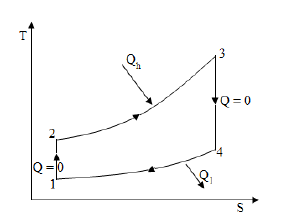Solution:

it is clear from the diagram 1-2: isentropic compression  2-3: isobaric or isochoric heat addition 3-4: isentropic expansion 4-1: isobaric or isochoric heat rejection So it must be Otto or Brayton cycle. ‘Otto’ option is not there so answer is Brayton cycle

QUESTION: 27

Assertion (A): Brayton cycle is not suitable for reciprocating engines.

Reason (R): A reciprocating engine cannot have complete expansion.

Solution:
QUESTION: 28

For air standard Brayton cycle, increase in the maximum temperature of the cycle, while keeping the pressure ratio the same would result in

Solution:

air standard efficiency of Brayton cycle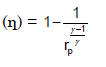QUESTION: 29

In a simple gas turbine power plant operating on standard Brayton cycle power needed to drive the compressor is 175 kW, rate of heat supplied during constant pressure heat addition process is 675 kW. Turbine output obtained during expansion is 425 kW. What is the rate of heat rejection during constant pressure heat rejection?

Solution: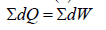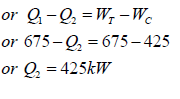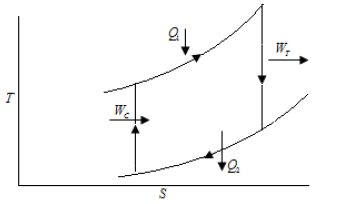QUESTION: 30

The given figure shows an open-cycle gas turbine employing reheating, on T-splane. Assuming that the specific heats are same for both air and gas, and neglecting the increase in mass flow due to addition of fuel, the efficiency is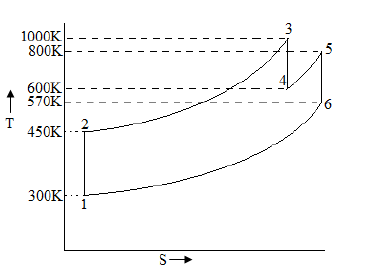Solution: